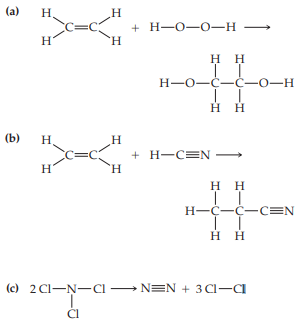×
Get Full Access to Chemistry: The Central Science - 12 Edition - Chapter 8 - Problem 69e
Get Full Access to Chemistry: The Central Science - 12 Edition - Chapter 8 - Problem 69e

×

# Bond Enthalpies (Section)Using Table estimate ?H for eachISBN: 9780321696724 27

## Solution for problem 69E Chapter 8

Chemistry: The Central Science | 12th Edition

• Textbook Solutions
• 2901 Step-by-step solutions solved by professors and subject experts
• Get 24/7 help from StudySoup virtual teaching assistantsChemistry: The Central Science | 12th Edition

4 5 1 257 Reviews
27
0
Problem 69E

BOND ENTHALPIES (section 8.8)

Using Table estimate ΔH for each of the following gas-phase reactions (note that lone pairs on atoms are not shown):Step-by-Step Solution:
Step 1 of 3

PSYCH STATS POWERPOINT CHAPTER TWO ● frequency:​ the number of times a score occurs ● distribution​: shape of scores ● frequency distribution​: organized display of scores ○ descriptive stats ● tables: simplest format ○ lists every score and frequency ● graphs ○ visual depiction ○ many types Frequency Distribution Table ● find the largest value, write it in the table ● find each time it occurs, record frequency ● repeat ● sum of all scores ○ take each individual score from table ○ multiply each score by the frequency ● proportion: f/n ● percentage: (f/n)100 ● the sum of all the total number of frequencies: n ● if your data set has a large range: use a group frequency table

Step 2 of 3

Step 3 of 3

##### ISBN: 9780321696724

Unlock Textbook Solution